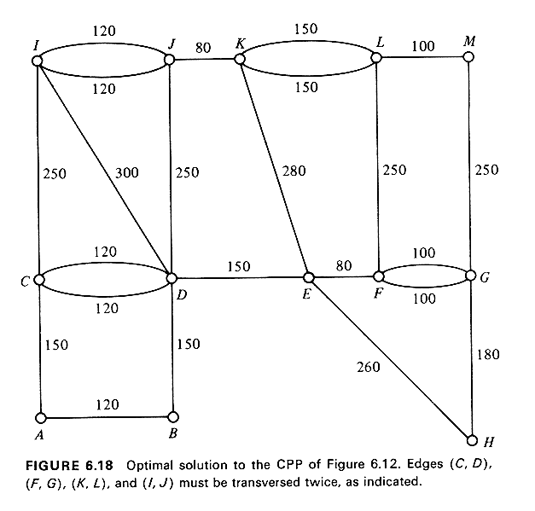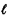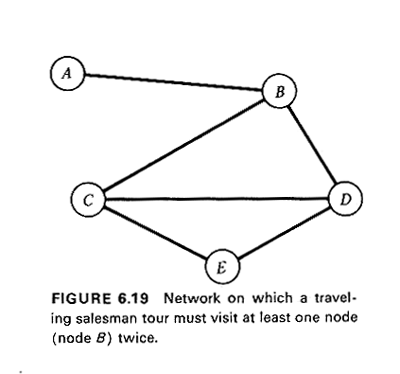### 6.4.5 Node Covering: The Traveling Salesman Problem

When deliveries, collections, or visits must be made to (or from) a number of specific (and, often, widely separated) points, the routing problem that must be solved becomes a node-covering one. The demand (or supply) points can then be represented as the nodes on the network model of the urban transportation grid and the question of the order in which to visit these nodes so as to achieve some objective is then addressed.

The most fundamental and best known of all node-covering problems is the traveling salesman problem (TSP):

Find the minimum distance route that begins at a given node of a network, visits all the members of a specified set of nodes on the network at least once, and returns eventually to the initial node.This staternent of the TSP is more general than the one most commonly used. The latter specifies that each node must be visited exactly once. However, this implicitly assumes that it is possible to find a tour with such a property. This is not, for instance, the case in the network of Figure 6.19, where in order to visit node A, one must visit node B twice.

We shall be particularly interested in solving the TSP in the following context: n--1 points must be visited by a vehicle which must begin and finish its tour at another specific point (depot). The transportation network that interconnects these n (in total) points is completely connected, that is it is possible to go directly from any point to any other point without passing through any of the other points in the set. The shortest distances between all pairs of points are equal to the lengths of the direct links between the two points, i.e. if i and j are any two of the n points then d(i, j) =(i,j). This implies that our network (or travel metric) satisfies the triangular inequality(i, j) <(i, k) +(k, j) for any three points i, j, and k. finally, the shortest distance matrix is assumed to be symmetric.The applicability of this version of the TSP to continuum models and to network models of the urban environment is clear.13 The complete connectivity of the network and the triangular inequality assure us that a shortest length traveling salesman tour through the n points can be found such that each point is visited exactly once.

That the TSP may be a very difficult problem to solve can now be seen by the fact that in our (simplified) version of the TSP there are (n - 1)! different orderings of the points to be visited, that is, (n - 1)!/2 different solutions of the TSP (since each tour can be run in either of two directions). Thus, a TSP with only 10 nodes has 1,814,400 possible solutions, and one with 20 nodes about 1.2 x 1018.

The TSP has indeed turned out to be an extremely difficult problem. While several exact algorithms (i.e., algorithms that are guaranteed to terminate with an optimal solution) have been devised for the TSP over the years, none of them is efficient, in the sense of being a polynomial-time algorithms. In fact, the TSP is considered b; mathematicians and computer scientists as a problem which is so difficult intrinsically that no efficient algorithm will probably ever be found to solve it.14 (On the other hand, no one has yet proven that no efficient algorithm for the TSP exists, so there is still hope!)

Of the existing exact algorithms, an early one [HELD 62] based on the idea of dynamic programming is O(n22n), where n is the number of points in the tour. As if this were not bad enough, the algorithm also requires computer storage proportional to n2n. Thus, even the largest available computers can handle problems with only up to about 15 points when this algorithm (which, of course, is so much better than exhaustive enumeration) is used. What is now generally considered as one of the "best" exact algorithms available [HELD 71] becomes impractical with problems involving more than about 70 points.15

Is our version of the TSP (symmetric distance matrix, complete connectivity, triangular inequality) any easier than a general TSP (e.g., one for which the distance matrix can be asymmetrical as in most street networks with one-way streets) ? The answer, in principle, is unfortunately "no.,, It has been shown [PAPA 77] that the two problems are equally difficult in the sense of NP-completeness. That is, if an efficient exact algorithm can be found for TSP1(which is how we shall denote our simplified version of the TSP from now on), an efficient algorithm must also exist for the general TSP, as well.

Be that as it may, it is still true that the intuitive appeal of TSP1 greatly facilitates the construction of heuristic algorithms which lead to good, if not necessarily optimal, solutions of these problems. In recent years a few fine such algorithms have been devised which solve quite large TSPl,s at very reasonable computational costs [LIN 73, CHRI 76]. Moreover, it is often possible to use such heuristics to obtain manually good solutions to modest-size TSP1's.

The emphasis on good heuristic solutions is also justified by the fact that, in practice, data inaccuracies and the probabilistic nature of such things as travel times between points make the concept of an "optimal" solution a rather theoretical one.

In the next section we shall review in detail one interesting and well-performing heuristic algorithm. In the process we shall have occasion to discuss a couple of properties of optimal tours for Euclidean TSP1's (i.e., TSP1's with Euclidean travel metrics) that further aid the manual solution of these latter problems. A good review of many other TSP heuristic algorithms and comparisons of their performance can be found in [GOLD 79].

13 Note that this also includes directed networks (i.e., cases with one-way streets) as long as, for the n points of interest, d(i,j) = d(j,i).

14 The TSP is the prototypical one of the special class of difficult combinatorial problems called NP-complete problems.

15To our knowledge, the largest problem that has been solved using this method has 121 points (KNUT 76). However, that problem has a special structure that considerably facilitates its solution.